Tutorials / Modeling Venture Debt for Startups

# Modeling Venture Debt for Startups

## Introduction

Venture debt is a popular option for startups looking to extend their runways between priced equity rounds. In an unfavorable equity financing environment (e.g. during a recession), founders use venture debt to secure working capitals for scaling while avoiding dilutions at highly undesirable valuations.

Modeling venture debt using spreadsheet could prove onerous and prone to human errors. With Pry's modeling tools, you can model venture debt with ease and clarity. Today, we will walk through a typical venture debt schedule and how you can set it up in Pry.

This tutorial will use a blank Pry workspace to demonstrate how the venture debt model is flown to our financial statements. Afterward, you can reference our setup guide to learn about importing data to Pry and our financial projections guide to finishing your complete financial plan in Pry.

The venture debt in this tutorial will be in the form of growth capital, a term loan, the type of venture debt that startups take on most often.

## Creating assumptions

One significant difference between venture debts and our regular term loans is that venture debts usually have an interest-only period, during which the borrowers would only pay the interests on the loans without paying down the principal.

Here is an example of what a venture debt's terms would look like, and we will use these terms to create our model:

• Principal amount (amount borrowed): \$2,000,000
• Annual interest rate: 12%
• Loan term: 3 years
• Payments per year: 12
• End of period payment or beginning of period payment: End of period payment
• Interest-only payments: 6
• Loan origination date (payment start date): July 2022

Let us create a new model and name it "Venture Debt." To create a new model, go to the "Models" page by clicking on "Models" in the top right corner of your Pry workspace.Then, in the top right-hand corner of our workspace, below our workspace name, we will find a model named "Revenue" pre-populated for you, and next to the model's name, there is a dropdown arrow. Click on the dropdown arrow, and we will find a "+" sign to add a new model. Proceed to click on the "+" sign, and we will be redirected to this new model's page. Now, click on the dropdown arrow next to the new model's name (temporarily named "New Model"), hover over the new model, click on the three vertical dots that appear on hover, and select the first option, "Rename Model." Next, type in "Venture Debt" and hit enter.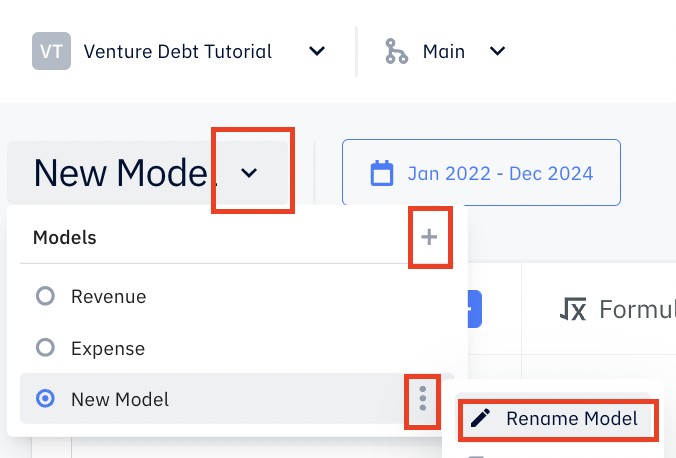We will create inputs in the Inputs column corresponding to the venture debt terms mentioned above in the new venture debt model.

To create an input, click on the "+" in the Inputs column and pick the appropriate type for each of our assumptions.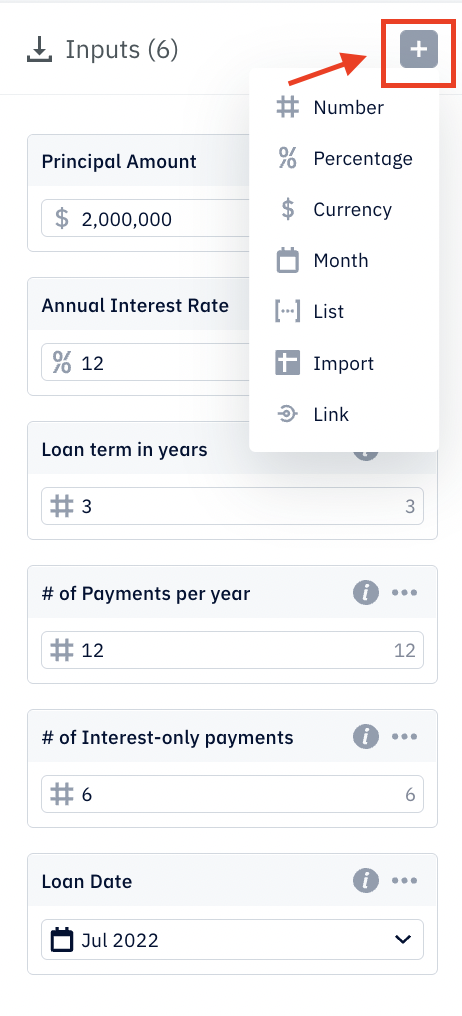As we see in the screenshot above, we should have four inputs created for this model corresponding to the assumptions we made in the beginning. With this, we are ready to start modeling the venture debt. First, we will calculate the monthly loan payments for the initial interest-only payment periods and the payments for the remaining periods where principals will be paid down.

## Setting up on Loan Origination Date.

On the Loan Origination Date or the day when we receive the loan from venture debt, we need to put it on the Liabilities account on the Balance Sheet and also record an increase in cash balance on the Assets side of the Balance Sheet (in Pry's built-in version of the Balance Sheet, cash is called Banks). Setting this up in Pry is very simple. We only need one formula adding the increase of Liabilities balance of \$2,000,000 at the Loan Origination Date.

To create this formula, click on the "+" sign in the Formulas column, and a new formula box will appear with the formula box's name highlighted. We will rename this formula "Loan to Balance Sheet." And in the formula box, type in the following shown in the screenshot: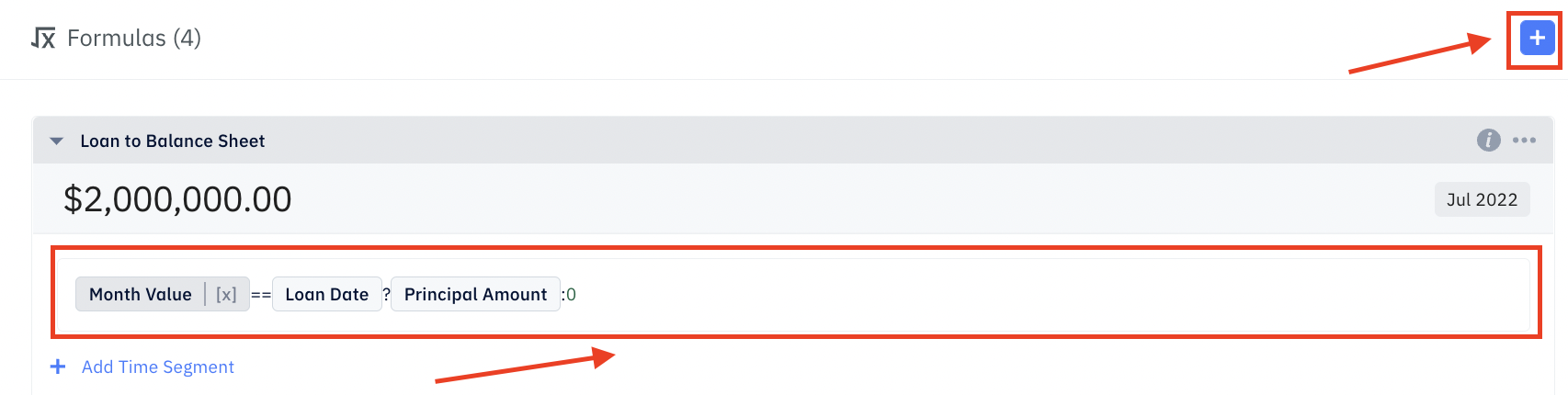This formula will return \$2,000,000 for July 2022, the Loan Origination Date, and zero for other months. To familiarize yourself with Pry's built-in functions and variables, you can reference the doc page here.

To validate this formula's outputs, we can click on the shaded box within the formula box that says "Jul 2022" and change it to a different month and see if the output is zero.

Now, we will link the outputs of the formula to the accounts on our Balance Sheet. To link the outputs, we need to create output by clicking on the "+" sign in the Outputs column. An output box will appear. Select the formula we just made, "Loan to Balance Sheet" in the first dropdown and Liabilities in the second dropdown. After adding the \$2,000,000 to the Liabilities account on the Balance Sheet, a \$2,000,000 cash balance increase (shown in the Banks account on the Assets side) is automatically generated to balance.

We can go to the Financials page to check. Once we are on the Financials page, find the dropdown arrow next to Financial Report, click on it, and select Balance Sheet. We will discover both Assets and Liabilities accounts are showing a \$2,000,000 balance starting July 2022.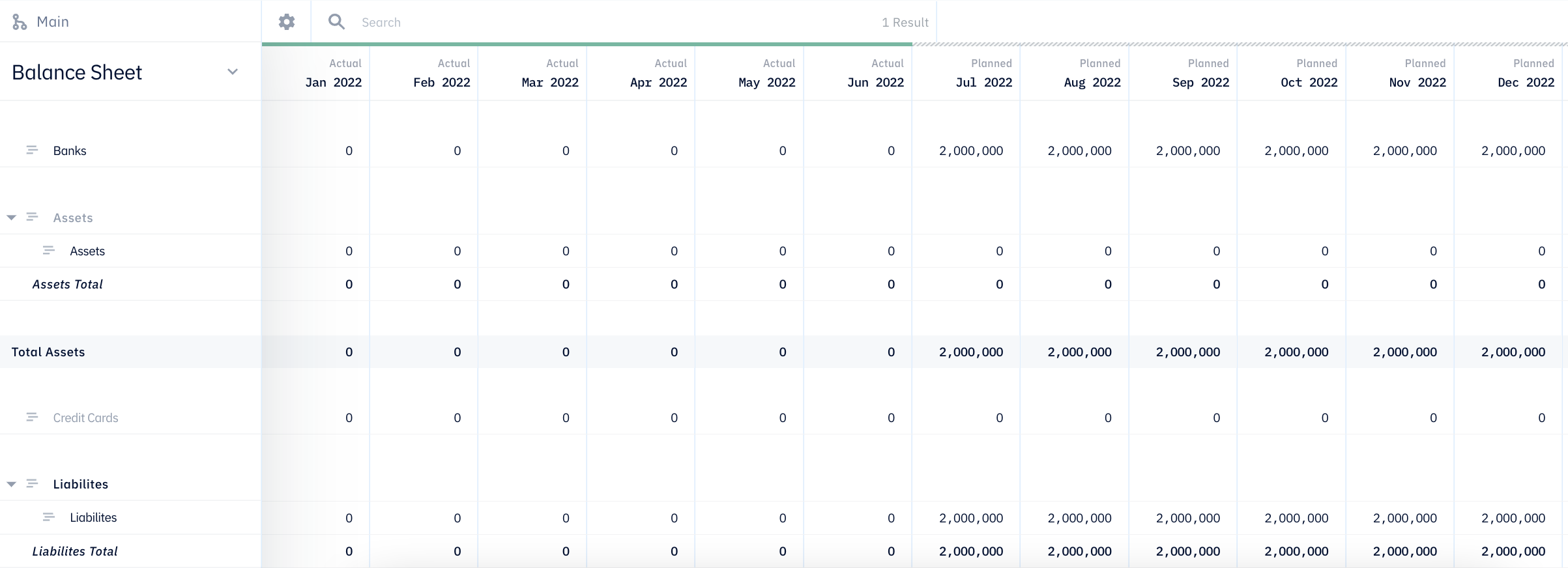Then, we will calculate the payments for interest-only periods and the remaining period and link them to the appropriate financial accounts.

## Calculating monthly payments

### Interest-only payments

Interest-only payment per its namesake is a payment where the borrower only pays the interest amount based on the amount borrowed, a.k.a. principal amount. Interest-only payments for venture debt typically happen only at the beginning of the loan term.

For this fictional venture debt, the interest-only period is six months, meaning we will only need to pay the principal amount times the interest rate for the first six monthly payments. The interest rate for monthly payments is calculated using the annual interest rate divided by the payment periods per year). To translate this into a formula, we have:

\$2,000,000 Principal Amount * (12% Annual Interest Rate /12 Payments per year) = \$20,000 per payment

And there will be six such monthly payments starting on July 2022. So from July 2022 to December 2022, we would like our model formula to return \$20,000 every month, and zero for other months. To create a formula using this logic in Pry, we will need to create a new formula by clicking on the "+" sign in the Formulas column. A new formula will popup and its name will be highlighted, we can change it to "Interest-only Payments". In the white space in the formula box, let us type in the following formula as show in the screenshot below, which will give the exact outputs as described above.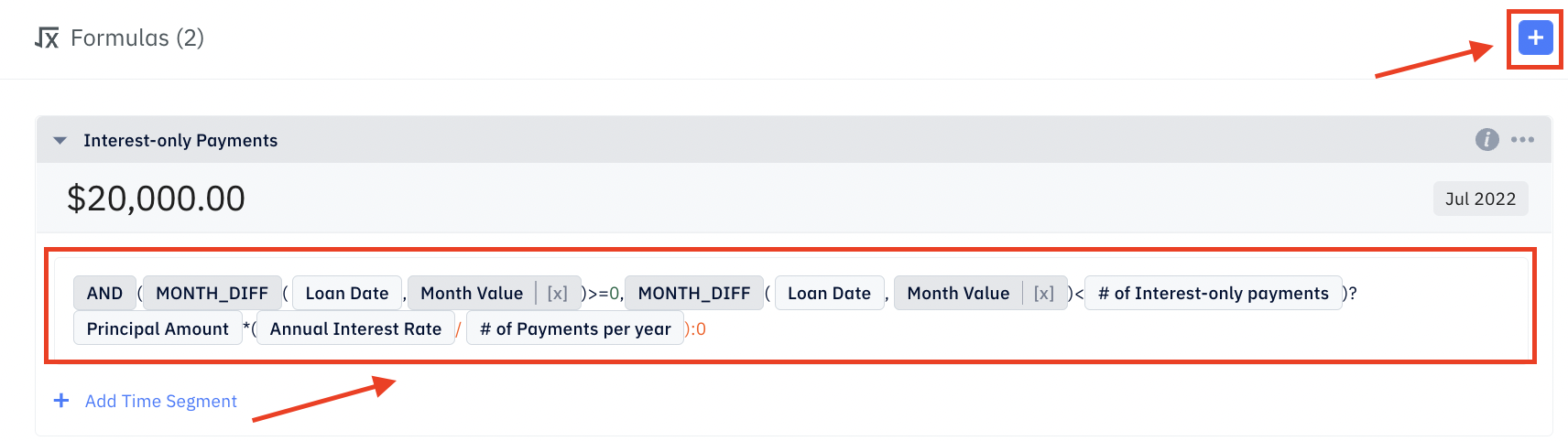You can refer to our docs for the built-in variables and functions used in this formula, such as Month Value and If/Then/Else.

As a check, try clicking on the shaded box with the date "Jul 2022" in it (as shown in the screenshot above) and change it to a different date to see what the formula returns.

Now, since the interest-only payments are the expenses that incur from taking on the venture debt, they should go into the Interest Expense account under the Expense portion of your Income Statement. (Pry's Financial Report includes a Net Income section, which has the accounts and structure of a typical Income Statement. We can also automatically generate Income Statement report, called Profit & Loss report in Pry, using Pry's custom report feature after the Financial Report is populated.) To categorize these payments as Interest Expense, we will link the outputs of the Interest-only Payment formula to our Financial Report. Click on the "+" in the Outputs column, an output box will appear. In the first dropdown, select the Interest-only Payments we just created. In the second dropdown, select Interest Expense.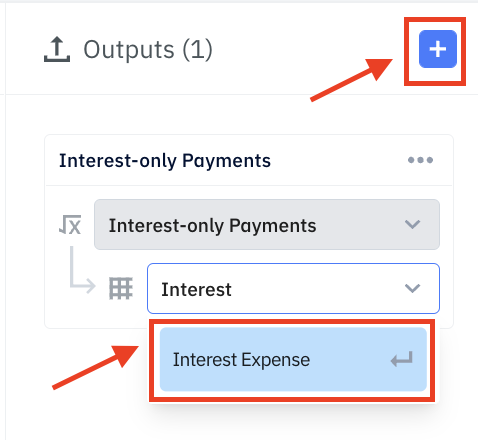We can then check by going to the Financials tab and scroll down to Financial Report. We will find the Interest Expense account under Expense showing -\$20,000 each month from July 2022 to December 2022.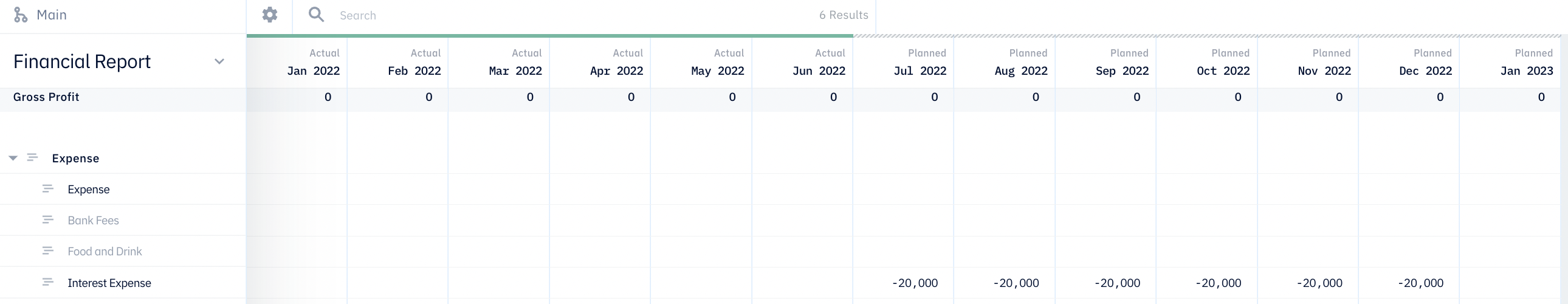Next, we will calculate the monthly payments for the rest of the loan term.

### Payments for the remaining loan term

After the initial six months of interest-only payments, each of the remaining monthly payments will be a mix of the interest that occurred on the principal amount and a portion of the principal amount. All the monthly payment amounts are the same. And at the end of the three-year loan term, the principal amount will be zero. Let us first calculate the monthly payment amount.

To calculate the monthly payment, we can use the formula for a typical term loan (e.g. fixed-rate mortgage).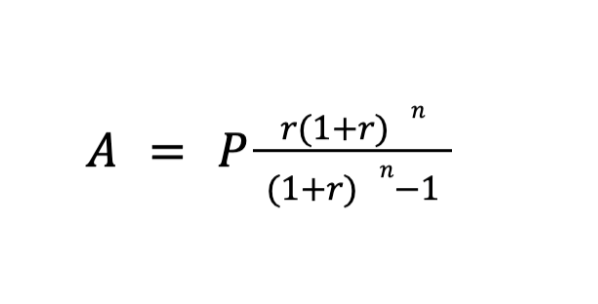• A represents the monthly payment amount, the amount we are solving for;
• P represents the principal amount, \$2,000,000;
• r represents the interest rate per each payment period, which can be calculated using the annual interest rate divided by the number of the payment period periods per year, 12%/12 =1%;
• n represents the loan term in payment; in this case, it should be the remaining loan term, 3 years * 12; payment periods per year - 6 interest-only payment periods = 30 payment periods;

Putting everything together, the A, or monthly payment, equals \$77,496.23. Then, we will create a formula that returns \$77,496.23 for 30 months starting January 2023 and ending June 2025. To do this in Pry using the inputs we created, create a new formula and name it "Payments after interest-only periods" and type in the following the syntax shown in the screenshot below: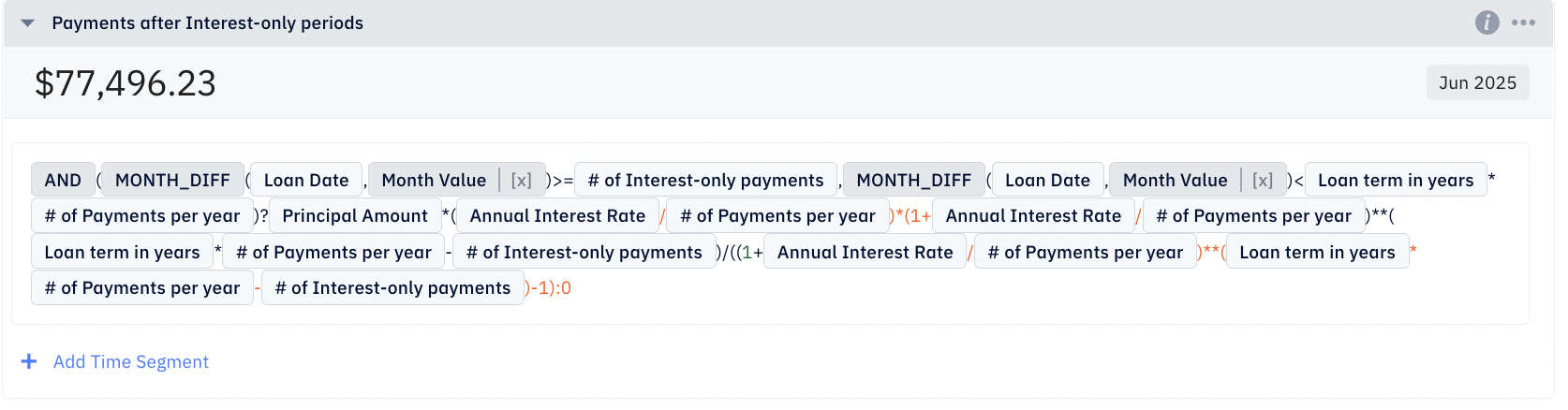The syntax for exponentiation is **. You can check out the list of basic syntax operators here.

We can check by clicking on the shaded box with a date in it and changing it to a different date, and seeing if the formula returns the expected amount.

Since we now know the monthly payment amount, we can calculate each payment's interest and principal portions and allocate them to the appropriate financial statement accounts.

We will create three formulas in Pry:

1. Principal amount owed, for tracking the remaining principal amount each period
2. Interest portion of payment after interest-only periods, for tracking Interest Expense on the Income Statement
3. Principal portion of payment after interest-only periods, for tracking principal paydown on the Balance Sheet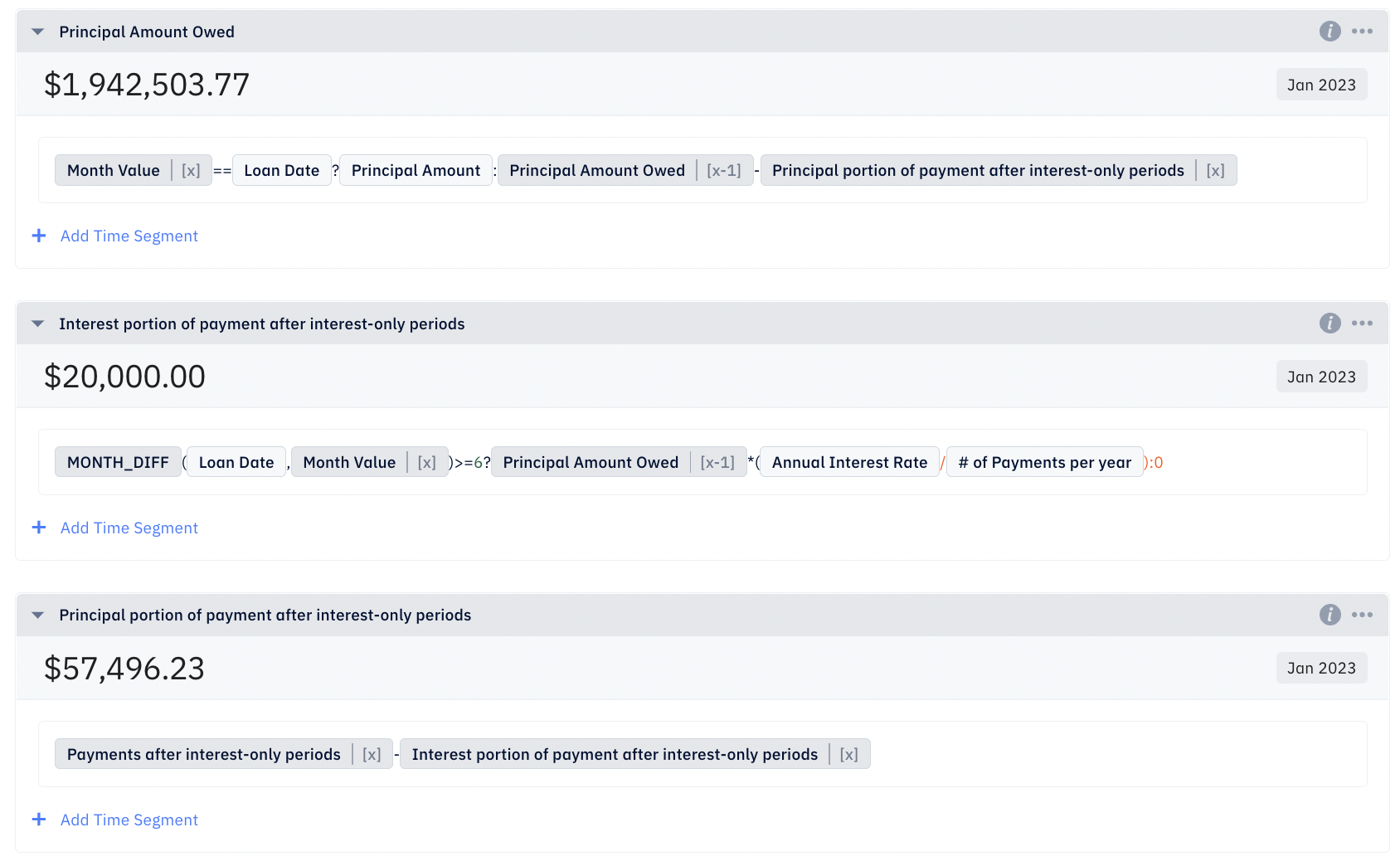The screenshot above shows the Pry functions and variables for each formula. We are using recursions here to call the previous month's output of one formula into another. Pry makes recursive formulas super easy by providing you with built-in time variables.

Once again, to check the outputs of the formulas, we click on the shaded box with the date in it and change the date to July 2025, the month after the last payment. Again, we will find all three formulas return zero.

Lastly, let us link the outputs to the appropriate accounts on our financial statements.

Create two outputs:

• For the first one, select the formula "Interest portion of payment after interest-only periods" in the first dropdown and "Interest Expense" in the second dropdown
• For the second one, select the formula "Principal portion of payment after interest-only periods" in the first dropdown and "Liabilities" in the second dropdown. This will reflect the decrease in liabilities as we pay down the loan.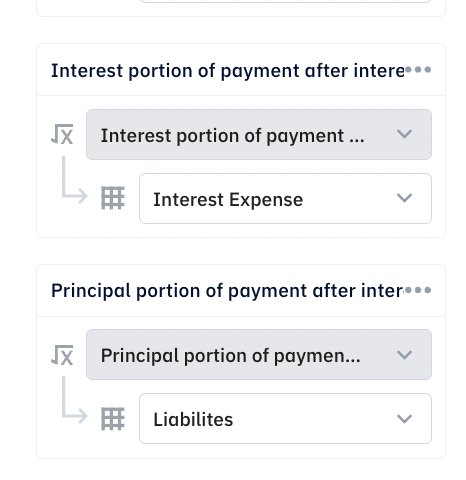Finally, let us go back to the Financials page and check the Expense section on the Financial Report. We will find the Interest Expense account showing decreasing amounts until the amount reaches zero in July 2025.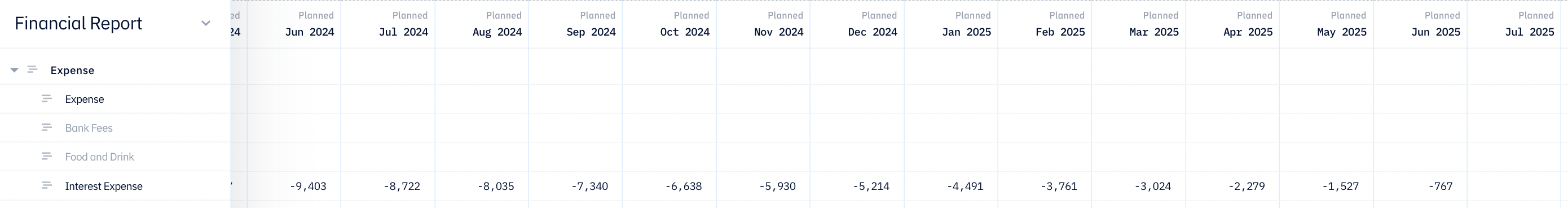Then, we will switch to the Balance Sheet and check the Assets and Liabilities balances. We will find the Assets balance keeps decreasing until it reaches -\$444,887 in June 2025, the last month in the loan term. -\$444,887 is the total amount of interest incurred for the loan term. Likewise, the Liabilities balance keeps decreasing until it reaches zero in June 2025.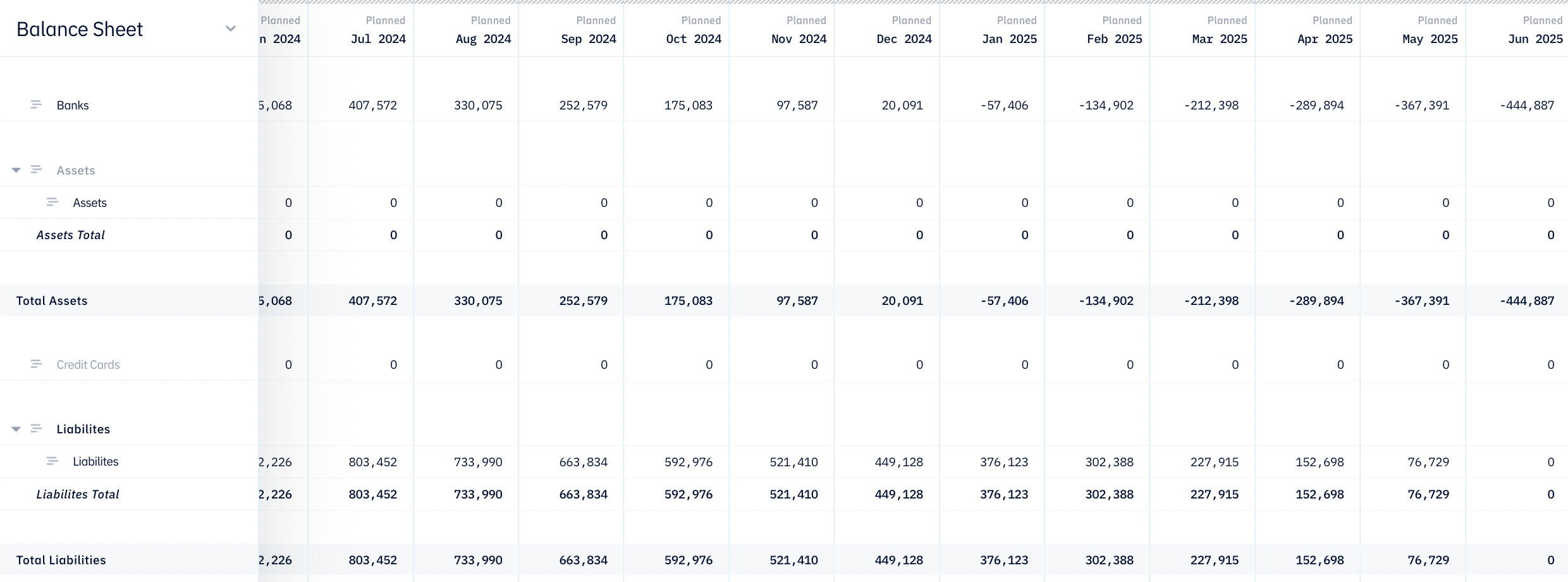We have now completed the venture debt tutorial for startups. This example represents a majority of venture debt terms for startups we see nowadays. You can also recycle this model for a traditional loan by setting the interest-only period to zero.

#### Ready to get started?Replace spreadsheets and get transparent financial projections with Pry.

© Pry Financials Inc.